Perform logistic regression using TensorFlow

In this tutorial, learn how to create a Jupyter Notebook that contains Python code for defining logistic regression, then use TensorFlow (tf.keras) to implement it. The Notebook runs on IBM Cloud Pak® for Data as a Service on IBM Cloud®. The IBM Cloud Pak for Data platform provides additional support, such as integration with multiple data sources, built-in analytics, Jupyter Notebooks, and machine learning. It also offers scalability by distributing processes across multiple computing resources. You can choose to create assets in Python, Scala, and R, and use open source frameworks (such as TensorFlow) that are already installed on the IBM Cloud Pak for Data as a Service platform.

The difference between linear and logistic regression

While linear regression is well suited for estimating continuous values (for example, estimating house prices or product sales), it is not the best tool for predicting the class in which an observed data point belongs. To provide estimates for classification, you need some guidance on what would be the most probable class for that data point. For this, you would use logistic regression.

Linear regression

Linear regression finds a function that relates a continuous dependent variable, y, to some predictors (for example, independent variables x1 or x2). Simple linear regression assumes a function of the form:

y = w0 + w1 x x1 + w2 x x2 + ...

It finds the values of w0, w1, and w2. The term w0 is the intercept or constant term (it’s shown as b in the following formula):

Y = W X + b

Logistic regression

Logistic regression is a variation of linear regression and is useful when the observed dependent variable, y, is categorical. It produces a formula that predicts the probability of the class label as a function of the independent variables.

Despite the name logistic regression, it is actually a probabilistic classification model. Logistic regression fits a special s-shaped curve by taking the linear regression and transforming the numeric estimate into a probability with the following function:

𝑃𝑟𝑜𝑏𝑎𝑏𝑖𝑙𝑖𝑡𝑦𝑂𝑓𝑎𝐶𝑙𝑎𝑠𝑠=𝑡ℎ𝑒𝑡𝑎(𝑦)=𝑓𝑟𝑎𝑐𝑒𝑦1+𝑒𝑦=𝑒𝑥𝑝(𝑦)/(1+𝑒𝑥𝑝(𝑦))=𝑝

This produces p-values between 0 (as y approaches minus infinity) and 1 (as y approaches plus infinity). This now becomes a special type of non-linear regression.

In this equation, y is the regression result (the sum of the variables weighted by the coefficients), exp is the exponential function, and theta(y) is the logistic function, also called logistic curve. It is a common “S” shape (sigmoid curve), and was first developed for modeling population growth.

You might have seen this function before in another configuration:

𝑃𝑟𝑜𝑏𝑎𝑏𝑖𝑙𝑖𝑡𝑦𝑂𝑓𝑎𝐶𝑙𝑎𝑠𝑠=𝑡ℎ𝑒𝑡𝑎(𝑦)=𝑓𝑟𝑎𝑐11+𝑒−𝑦

So, logistic regression passes the input through the logistic/sigmoid function, but then treats the result as a probability.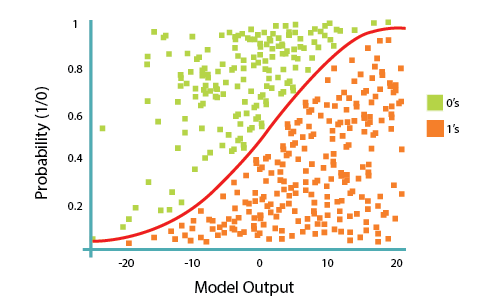Prerequisites

The following prerequisites are required to follow the tutorial:

Estimated time

It should take you approximately 60 minutes to complete this tutorial.

Steps

Step 1. Create IBM Cloud account

2. Search for Watson Studio.
3. Create the service by selecting a region and pricing plan.4. Click Create.Step 2. Create a new project

1. Click Get started to start the Watson Studio service.

2. Click Create a project, and create an empty project.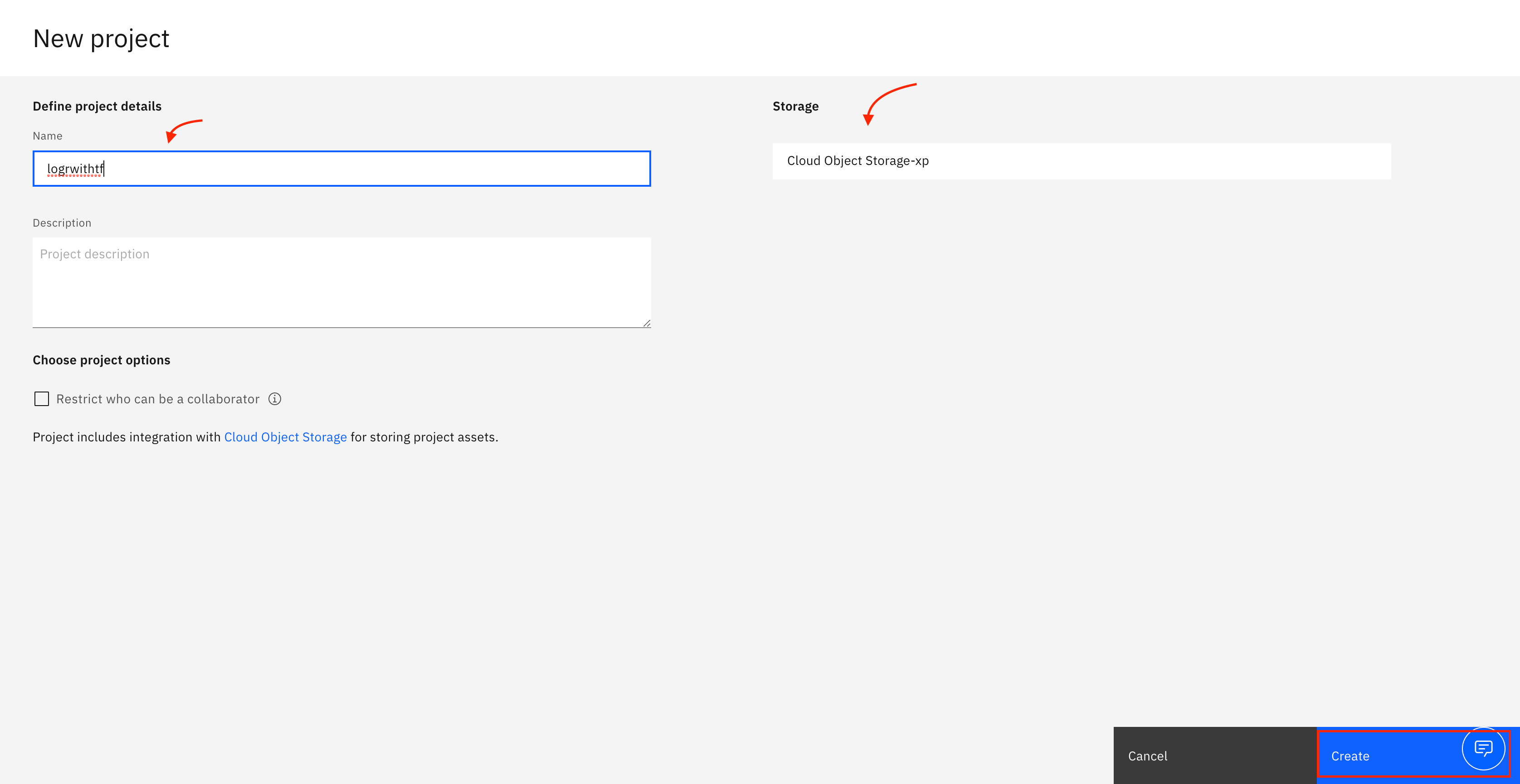4. Click Create. After your project is created, you are directed to a project dashboard.

Step 3. Associate the Watson Machine Learning Service with the project

1. Click the Settings tab.
2. Scroll down to Associated services, and click Add service.3. Select Watson in the drop-down menu.

4. Select Machine Learning.5. Click Associate service.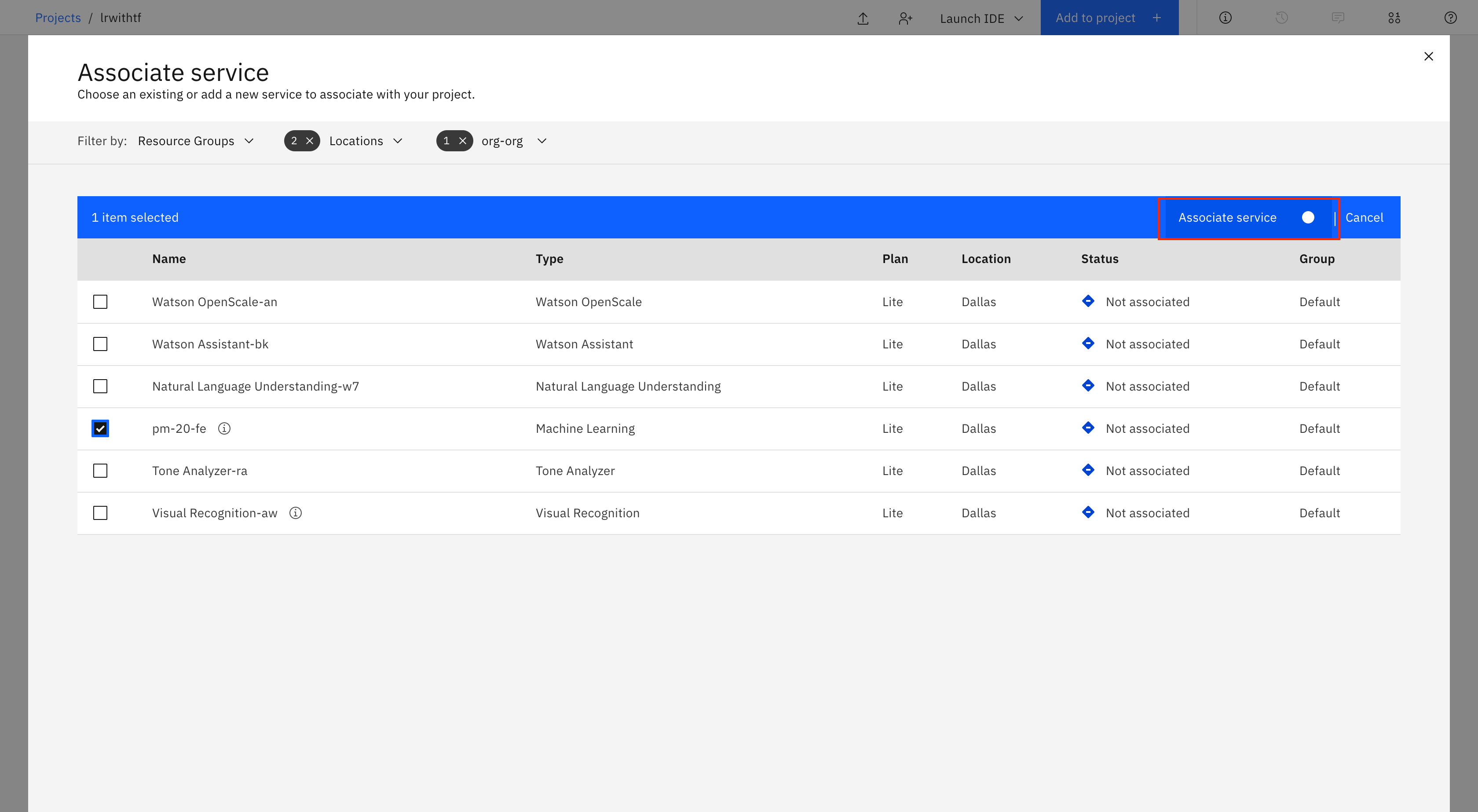Step 4. Add Notebook to project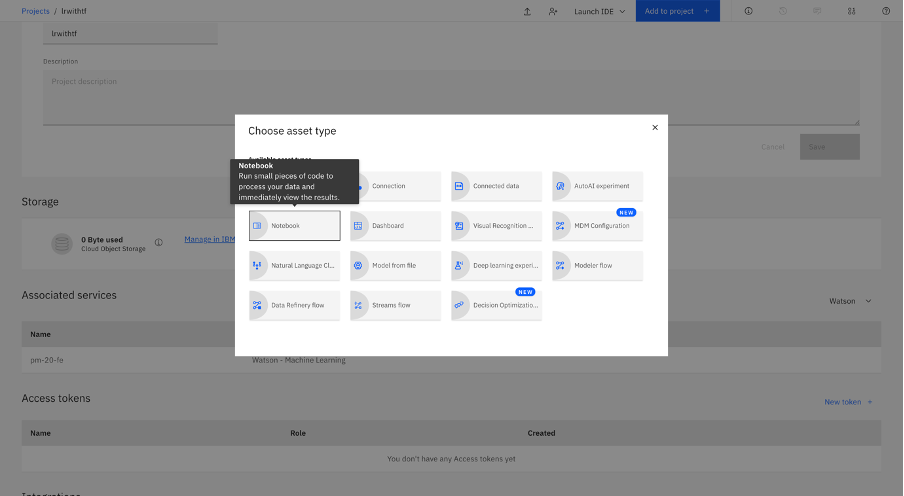2. Select From URL, and enter the following URL in the Notebook URL field.

https://github.com/IBM/dl-learning-path-assets/tree/main/fundamentals-of-deeplearning/notebooks/Logistic_Regression_with_TensorFlow.ipynb

3. Name your Notebook, and click Create.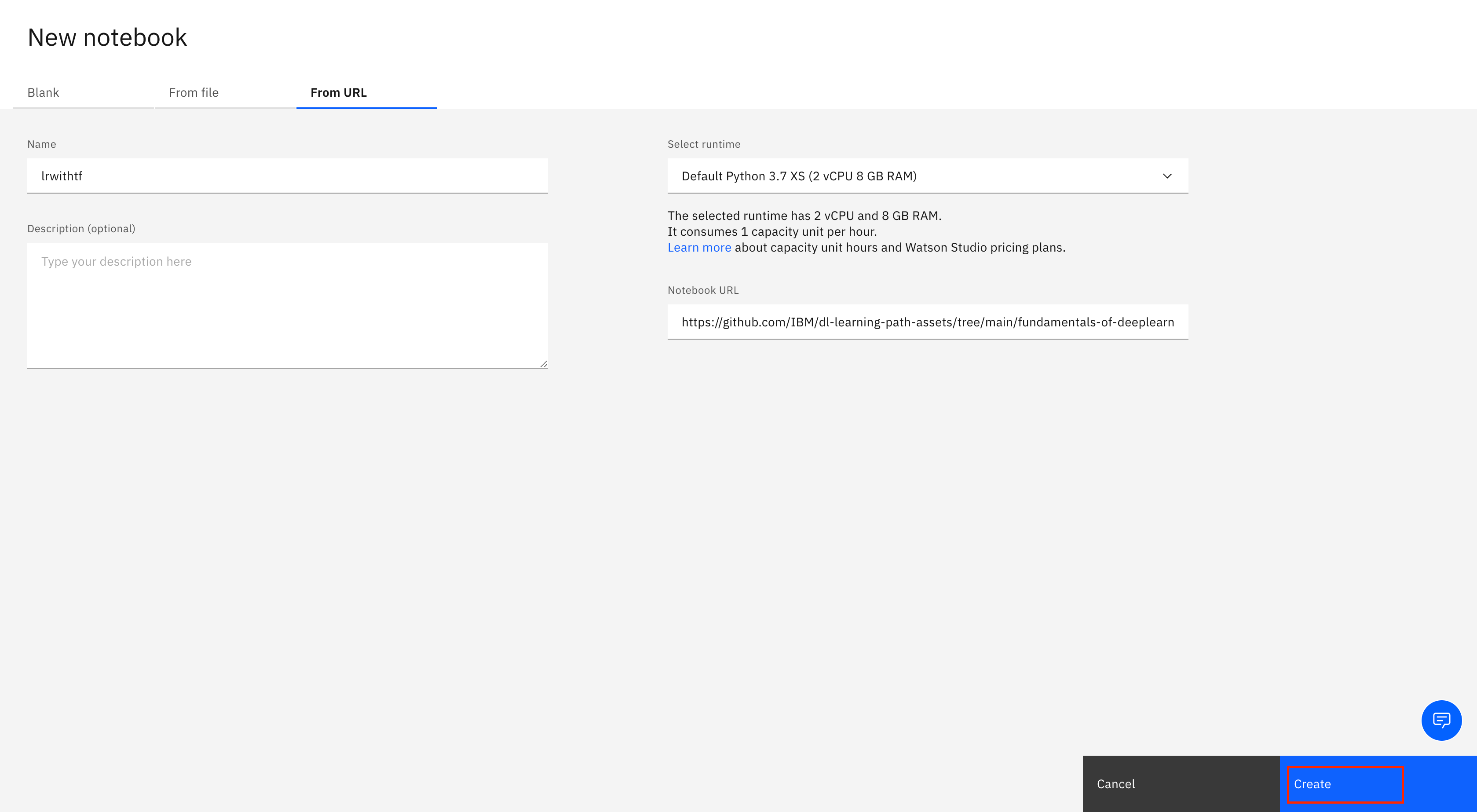Step 5. Run the Notebook

After the notebook is loaded, click Cell, then select Run All to run the Notebook.